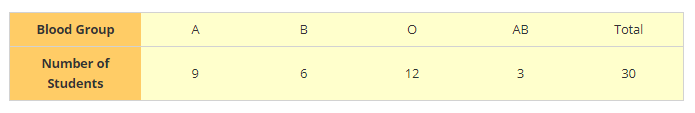# The Blood group table of 30 students of class IX is recorded as follows:

Question:

The Blood group table of 30 students of class IX is recorded as follows:

A, B, O, O, AB, O, A, O, B, A, O, B, A, O, O

A, AB, O, A, A, O, O, AB, B, A, O, B, A, B, O

A student is selected at random from the class from blood donation. Find the probability that the blood group of the student chosen is:

1: A

2: B

3: AB

4: O

Solution:1. Probability of a student having blood group A

$=\frac{\text { Favorable out come }}{\text { Total out come }}$

= 9/30

= 0.3

2. Probability of a student having blood group B

$=\frac{\text { Favorable out come }}{\text { Total out come }}$

= 6/30

= 0.2

3. Probability of a student having blood group AB

$=\frac{\text { Favorable out come }}{\text { Total out come }}$

= 3/30

= 1/10 = 0.10

4. Probability of a student having blood group O

$=\frac{\text { Favorable out come }}{\text { Total out come }}$

= 12/30 = 0.4